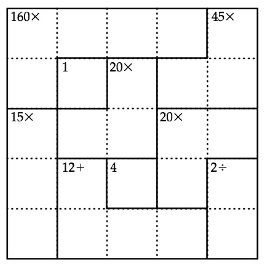Chapter 1.1, Problem 5EEMathematical Excursions (MindTap C...

4th Edition
Richard N. Aufmann + 3 others
ISBN: 9781305965584

Solutions

Chapter
SectionMathematical Excursions (MindTap C...

4th Edition
Richard N. Aufmann + 3 others
ISBN: 9781305965584
Textbook Problem

EXCURSION EXERCISESSolve each of the, following puzzles. Note: The authors of this textbook are not associated with the KenKen brand. Thus the following puzzles are not official KenKen puzzles; however, each puzzle can be solved using the same techniques one would use to solve an Official KenKen puzzle.To determine

To solve: The given puzzle using Ken Ken puzzle technique.

Explanation

Given:

 160x 45x 1 20x 15x 20x 12+ 4 2÷

Calculation:

Since given puzzle is a 5x5 Ken Ken puzzle, so we fill the numbers 1, 2, 3, 4 and 5.

In the second row, second element should be filled with 1 and in the fourth row

third element should be filled with 4 respectively.

Therefore, we get

 160x 45x 1 1 20x 15x 20x 12+ 44 2÷

Now, in the fifth column the operation for last two elements is division and the target number is 2.

Therefore, we get

 160x 45x 1 1 20x 15x 20x 12+ 44 2÷ 2 1

Now, for the first two elements of fifth column and for the fourth element of second row the operation is multiplication and the target number is 45.

Therefore, we get

 160x 45x 3 1 1 20x 3 5 15x 20x 12+ 44 2÷ 2 1

Now, for the second element of fourth row and for the middle three terms of the fifth row the operation is addition and the target number is 12.

Therefore, we get

 160x 45x 3 1 1 20x 3 5 15x 20x 12+ 3 44 2÷ 2 4 3 2 1

Now, for the last two elements of third row and for the fourth element of fourth row the operation is multiplication and the target number is 20...

Still sussing out bartleby?

Check out a sample textbook solution.

See a sample solution

The Solution to Your Study Problems

Bartleby provides explanations to thousands of textbook problems written by our experts, many with advanced degrees!

Get Started

In Problems 45-51, solve each system of equations. 49.

Mathematical Applications for the Management, Life, and Social Sciences

Evaluate the definite integral. /3/3x4sinxdx

Single Variable Calculus: Early Transcendentals, Volume I

Find the derivative. Simplify where possible. 41. y=cosh1x

Single Variable Calculus: Early Transcendentals